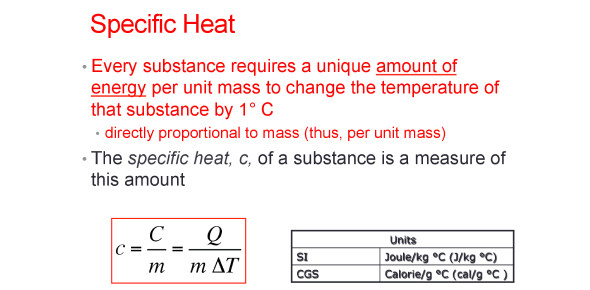# What Do You Know About Specific Heat? Quiz

8 Questions | Total Attempts: 1188SettingsCreate your own Quiz.

• 1.
What is specific heat?
• A.

Heat from the sun.

• B.

The amount of heat needed to to raise the temperature at room temperature.

• C.

The amount of heat needed to raise the temperature of 1kg of some material.

• D.

The heat needed to restore room temperature.

• E.

None of the above

• 2.
What is the formula for specific heat?
• A.

Q=c+m

• B.

T=m+c xq

• C.

Q=cx m x t

• D.

T =mxn+x

• E.

None of the above

• 3.
The specific heat of water is ?
• A.

1 calorie/gram C=1.846joule/gram

• B.

1 calorie/gram C=4.186joule/gram

• C.

1 calorie/gram C=8.416joule/gram

• D.

1 calorie/gram=1.648joule

• 4.
What does the q stand for in the formula?
• A.

The heat lost

• B.

The heat that escapes from something

• C.

The heat absorbed or released

• D.

All of the above

• 5.
What does the c stand for in the formula?
• A.

The heat lost from the substance

• B.

The specific heat of the substance

• C.

The energy from the substance

• D.

None of the above

• 6.
What does the m stand for in thje formula?
• A.

The volume of the sample in liters

• B.

The volume of the sample in millimeters

• C.

The mass of the sample in grams

• D.

The mass of the sample in kilograms.

• 7.
What law does specific heat relate to?
• A.

Einstein-Debye

• B.

Dulong and Petit

• C.

Both of these laws

• D.

None of these laws

• 8.
What substance has the highest specific heat?
• A.

Water

• B.

Metal

• C.

Dirt

• D.

Sand

Related TopicsBack to top
×

Wait!
Here's an interesting quiz for you.